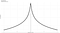# Bitcoin Daily Return Distribution (Part II)

## A probabilistic approach to Bitcoin trading (cont.)

In Part I, I estimated the daily percent returns of Bitcoin as Cauchy with scale 0.016 and location 0.0017. This enabled us to calculate the probability of a given daily event of profit or loss.

In this part of the series, I extend this approach to calculating the likelihood of a series of profits or loss events of a given size.

WARNING: This is not financial advice. Trading can get you REKT very quickly. Only do it if you know what you are doing and have fully comprehended all of the tax and legal obligations and sought the advice of a licensed financial advisor.

Here I assume each return is independent (no correlation to previous days profit or loss). An inspection of the scatterplot matrix of the lags reveals there is strong evidence to support this claim (figure 1).

Thus it is safe to assume each days profit is i.i.d. (when you see this, it means: independent and identically distributed) as Cauchy(s=0.016, l =0.0017). This leads us to be able to calculate the log-likelihood of a series of days of profits and loss.

Backup — whats log-likelihood? Further back — whats likelihood? Likelihood is simple the calculation of probability as it would be applied given the history of the data. Juxtaposed to when most people talk about probability: I think of it like this — probability is a question we seek to answer, but likelihood is like looking at the answer and trying to work out the question.

Log-likelihood is just what it sounds like — we take the natural log of the likelihood function. The likelihood function is the product of the data given its distribution. We know the distribution (Cauchy) and we know the data. Why do we take logs? Because the product of A and B is equal to the exponentiated sum of A and B, and adding stuff is much easier than multiplying it.

The density function of Cauchy is defined as

and the log-likelihood function for a sample of X of length n is

So now we can estimate the likelihood (and in particular — the magnitude of the likelihood) of a sequence of profit or losses.

For example — lets consider a day of 45% loss and a day of 20% profit following — l(0.0017,0.016;-0.45,0.2) = -5.74. Contrast this to the likelihood of a 45% loss and 20% loss following = -5.78.

Thus using this model I have shown that the first scenario is more likely than the second.

The most likely event (or maximum likelihood) is simply the location parameter (0.17%). Probably worth repeating: the most likely event given the history of bitcoin data on any daily return is a 0.17% profit.figure 2 — the log-likelihood distribution of the daily returns. The black line in the middle is the maximum likelihood estimate of 0.17%

In the next part of this series, I will extend this concept one step further — into simulations and forecasts.

Nick

Written by

Written by

## More From Medium

Welcome to a place where words matter. On Medium, smart voices and original ideas take center stage - with no ads in sight. Watch
Follow all the topics you care about, and we’ll deliver the best stories for you to your homepage and inbox. Explore
Get unlimited access to the best stories on Medium — and support writers while you’re at it. Just \$5/month. Upgrade